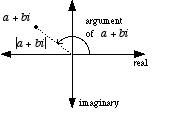index: click on a letter A B C D E F G H I J K L M N O P Q R S T U V W X Y Z A to Z index index: subject areas numbers & symbols sets, logic, proofs geometry algebra trigonometry advanced algebra & pre-calculus calculus advanced topics probability & statistics real world applications multimedia entrieswww.mathwords.com about mathwords website feedback

 Absolute Value of a Complex Number Modulus of a Complex Number The distance between a complex number and the origin on the complex plane. The absolute value of a + bi is written |a + bi|, and the formula for |a + bi| is $$\sqrt {{a^2} + {b^2}}$$. For a complex number in polar form  r(cos θ + isin θ)  the modulus is r.See also Real number, imaginary number, argument of a complex number